# Periodic point

(diff) ← Older revision | Latest revision (diff) | Newer revision → (diff)
A point on a trajectory of a periodic motion of a dynamical system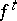(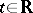or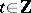) defined on a space, i.e. a point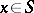such that there is a number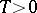for which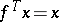butfor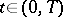. This numberis called the period of the point(sometimes, the name period is also given to all integer multiples of).
The trajectory of a periodic point is called a closed trajectory or a loop. When the latter terms are used, one frequently abandons a concrete parametrization of the set of points on the trajectory with parameterand considers some class of equivalent parametrizations: Ifis a continuous action of the groupon a topological space, a loop is considered as a circle that is topologically imbedded in; ifis a differentiable action of the groupon a differentiable manifold, a loop is considered as a circle that is smoothly imbedded in.
Ifis a periodic point (andis a metric space), then the-limit set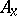and the-limit set(cf. Limit set of a trajectory) coincide with its trajectory (as point sets). This property, to a certain extent, distinguishes a periodic point among all points that are not fixed, i.e. if the space in which the dynamical systemis given is a complete metric space and if a pointis such that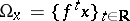, thenis a fixed or a periodic point of.